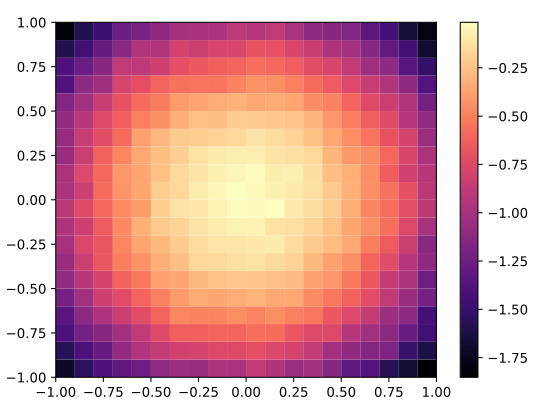A bare-bones Python library for quality diversity optimization.

## Efficient Illumination

pyribs is the official implementation of Covariance Matrix Adaptation MAP-Elites (CMA-ME) and other quality diversity optimization algorithms.

## Flexible Components

Every quality diversity (QD) algorithm in pyribs consists of three components.

``from ribs.archives import GridArchivearchive = GridArchive(    solution_dim=10,    dims=[20, 20],    ranges=[(-1, 1), (-1, 1)],)``

The Archive is a data structure that stores solutions generated by the QD algorithm.

``from ribs.emitters import EvolutionStrategyEmitteremitters = [    EvolutionStrategyEmitter(        archive,        x0=[0.0] * 10,        sigma0=0.1,    ) for _ in range(3)]``

Emitters generate new candidate solutions.

``from ribs.schedulers import Schedulerscheduler = Scheduler(archive, emitters)``

The Scheduler facilitates the interaction of the archive and emitters and acts as a scheduling algorithm for emitters.

## Intuitive Usage

pyribs components come together in an intuitive ask-tell interface inspired by pycma.

``import numpy as npfor itr in range(1000):    solutions = scheduler.ask()    # Optimize the 10D negative Sphere function.    objective_batch = -np.sum(np.square(solutions), axis=1)    # Measures: first 2 coordinates of each 10D solution.    measures_batch = solutions[:, :2]    scheduler.tell(objective_batch, measures_batch)``

## Insightful Visualizations

The pyribs visualize module integrates with Matplotlib to plot a heatmap of the archive after each experiment.

``import matplotlib.pyplot as pltfrom ribs.visualize import grid_archive_heatmapgrid_archive_heatmap(archive)plt.show()``## Easy Installation

pyribs supports Python 3.8 and above. The visualize distribution additionally installs visualization dependencies such as Matplotlib.

Distribution

Package

Command